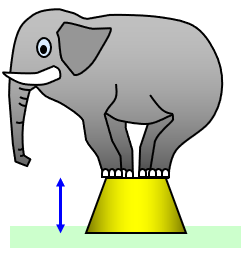# Potential energy

An object held up above the ground has potential energy
A coiled spring has potential energy
A stretched rubber band in a catapult has potential energy
A gas under pressure in a cylinder has potential energy
A bow pulled back ready to fire an arrow has potential energy
A spacecraft launched into orbit is given potential energy
Water held behind a dam or at the top of a waterfall has potential energy
The Earth's crust has potential energy is locked away in itIf an object is in a state where it could do work if released then we say that it has POTENTIAL ENERGY (sometimes written as p.e.). For this reason it can be called stored energy. You can think of potential energy that is "locked away" in an object and that can be used to do something when it is "let out". Just think of the energy released if a dam bursts or if the Earth's crust splits or bends as happens in an earthquake.

An object held above the ground has GRAVITATIONAL potential energy (G.P.E). A simpler word for this would be POSITION ENERGY.

We will look at gravitational potential energy a little more carefully. Now:

Work done = energy gained = force x distance moved.

Therefore to move a mass m up to a height (h) above the ground you will need to move a force F (= mg) through a distance h and so this needs mgh joules of energy. So if it takes that amount of energy to get it up then its gravitational potential energy is also mgh because it will release that energy when it falls to the ground.
(g is the strength oif the gravitational field = 10 N/kg on the Earth).

Gravitational potential energy = mass x g x height

When you lift up a 1 kg mass a distance of 1m you change 10 J of chemical energy in your body to 10 J of potential energy (ignoring heat for the moment). If you climb a steep mountain slope it is easier if you do it in a zig zag path. The gravitational potential energy that you gain is the same as if you had gone straight up but you gain it more slowly. The same also applies to driving a car up a mountain road – this is why they are built with so many bends, the car gains height slowly.

(There is some more information in the file called TRAINS See: 14-16/mechanics/Forces in motion/Problems/Trains)

Example problems
1. The potential energy of a 6 kg mass 4 m above the ground is:
Potential energy = 6x10x4 = 240 J

2. A 45 kg girl jumps from a 0.4 m high stool onto the ground. How much potential energy does she lose?
Potential energy lost = 45x10x0.4 = 180 J

3. A man slides a 25 kg box up a ramp onto the back of a lorry. If the ramp is 2 m long and the back of the lorry is 0.8 m above the ground how much potential energy does the box gain?
All that matters is the VERTICAL height moved and not the length of the ramp. So:
Potential energy gained = 25x10x0.8 = 200 J

You should realise that it does not matter which way the mass falls to the ground in example 1 or which way the box get to the back of the lorry in example 3. The potential energy change will be the same by whatever route.

A VERSION IN WORD IS AVAILABLE ON THE SCHOOLPHYSICS CD